1596427980

# Explanation of Quick Sort

In this article, I will be talking about quick sort & all the components that you need to know.

Quicksort is nothing but another algorithm to sort a list of items in ascending or descending order. To understand quick sort, we need to understand the following items:

• pivot
• how partition works
• recursion within quick sort & the base case

Pivot is the element that will be used to divide the list of values less than pivot to the left(or the right, if we are sorting it the other direction) and values greater than the pivot to the right of the pivot.

Let’s say we have the following:

``````/* Example array: 4 1 5 2 10 8 6 3 */

const array = [4,1,5,2,10,8,6,3];

//the first element,4, or the last element,3, is commonly used as a pivot in quick sort.

//after partition:
[?,?,3,?,?,?,?]
view raw
pivot.js hosted with ❤ by GitHub
``````

If 3 is chosen as the pivot, it will always land at where it should be left after a partition. In this case, that would be the 2nd index of the array.

To further demonstrate how that would work, view the following illustration: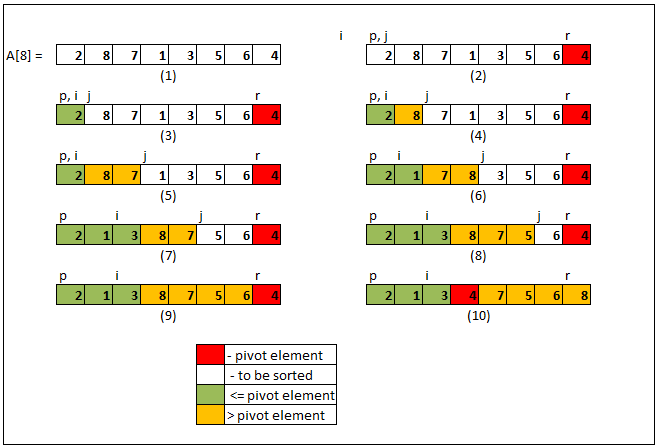On the illustration above, we have i, j, and the pivot. 4 is used as the pivot. i, initially, is set to -1. This indicates the number of elements we have that are less than the pivot. j, on the other hand, is used as an index to traverse through every element.

In partition, for every element j, if the pivot is great than arr[j], increment i and swap arr[i] and arr[j]. This will help put whatever element less than pivot on the left side of the array. J will increment regardless.

After the whole array is done, i equates to the number of elements that are less the pivot. Increment i by 1 and swap arr[i] with the pivot. Now the pivot is truly the dividing point between elements greater than it to the right and elements lesser than it to the left.

Afterward, quicksort will be called on the subarray to the left of the pivot and also to the right of the pivot. This process will repeat until there are no more subarrays to be sorted.

#sorting-algorithms #es6 #algorithms #javascript #web-development #algorithms

## Buddha Community1621251999

## Quick Flow Male Enhancement Reviews, Benefits Price & Buy Quick Flow?On the off chance that you fall in the subsequent class, Quick Flow Male Enhancement is the thing that your body is needing right now. The recently discovered male arrangement is the difficult solver for numerous types and types of erectile pressure causing brokenness and causes those issues to be rectified and henceforth blessings you with the more youthful sexual variant.

What is Quick Flow Male Enhancement?
With the new pill, you can supplant all extraordinary and numerous allopathic drugs you had been taking for each issue in an unexpected way. Quick Flow Male Enhancement is the one in all treating instrument pill and causes those explicitly hurtful issues to get right. Regardless of everything, those obstacles are restored and unquestionably, you can feel that the sexual peaks are better. This item builds body imperativeness and the measure of discharge that is required is likewise directed by it.

**How can it really function? **

Different results of this class have numerous regular Ingredients in them, yet the ones here in Quick Flow Male Enhancement are truly uncommon and furthermore natural in their reap and produce. This allows you to get the experience of the truth of more profound sex intercourse which you generally thought was a fantasy for you. Positively, this is a demonstrated natural thing, and relying upon it is no place off-base according to specialists. It is time that your body is given valuable minerals as requested by age.

It is fundamental that you visit the site and see by your own eyes you willing we are to help you in each progression. Start from the terms and furthermore know inconspicuously the states of procurement. Any question must be addressed as of now or, more than likely later things probably won’t go as you might suspect. Purchase Quick Flow Male Enhancement utilizing any method of online installment and you may likewise go for the simple EMI choice out there.

https://www.benzinga.com/press-releases/21/03/wr20313473/quick-flow-male-enhancement-reviews-fast-flow-male-enhancement-most-effective-and-natural-formul

#quick flow male enhancement #quick flow male enhancement reviews #quick flow male enhancement male health #quick flow male enhancement review #quick flow male enhancement offer #quick flow male enhancement trial1593542940

## Heap Sort Explained

As you may know, heapsort is also one of the most asked sorting algorithms besides mergesort and quicksort and today we’re going to talk about it and I’ll try my best to explain it in much simpler terms as for a beginner it can be quite complicated more so if one has less knowledge about heaps. But fear not I’ve got your back. So now let’s get into it.

Before moving on to heap sort you need to know about the following terminologies to properly understand the working of heapsort.

1. Heaps
2. Heap Property
3. Heapify

So let’s begin by explaining the terms stated above

# 1. Heaps

Heap data structure is a**_ complete binary tree that follows the heapify property_**. So what’s a complete binary tee you might ask?. A complete binary tree follows these conditions.

• All nodes in every level of the tree are filled except the leaf nodes.
• The nodes are as far left as possible.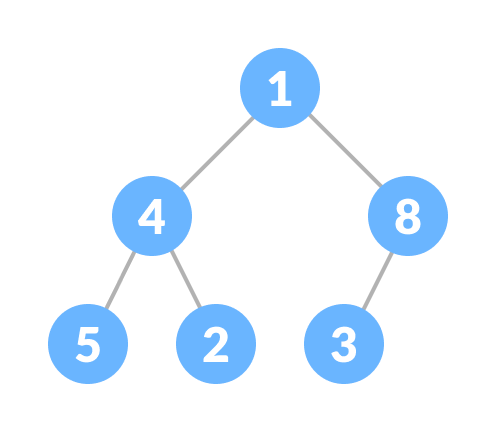Complete Binary Tree

The heap is shown below following the heap property and this is called Max heap. It has the same root and the child nodes but there is a slight difference. The Complete Binay Tree doesn’t care whether it’s root node is smaller or larger but it is of grave importance in case of the heap data structure.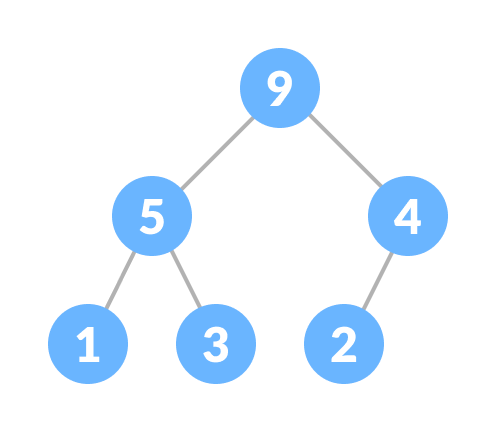Heap

# 2. Heap Property

While creating a heap it is essential to follow the heap property which states as follows:

• The heap where the parent node is always greater than the child nodes and provided that the root node is largest is called a Max-heap.Max Heap

• The heap in which the child node is always greater than it’s child nodes and the root node is the smallest node is called a Min-heap.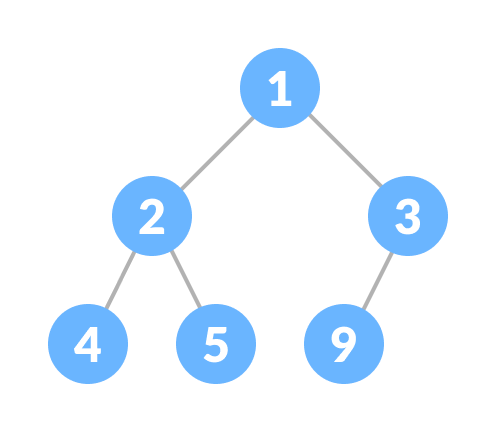Min heap

# 3. Heapify

Heapify is the** process of creating a heap data structure from a binary tree. **Now Heapify is the most important operation on a heap data structure as it helps to maintain the structure of the heap after several operations have been performed on the heap like(insertion, deletion). We will be using this operation a lot and it will come in handy while designing heap sort.

working of heapify is shown below

1. Select an input array.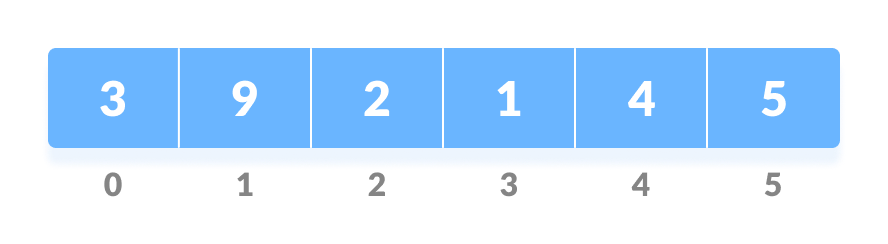Input array

2. Convert this array into a complete binary tree.

Complete binary tree created from the array

3. Create a heap from the binary tree.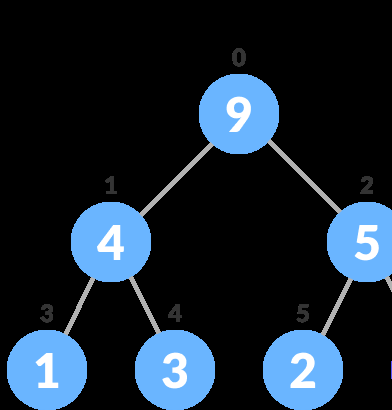After Heapify a max heap is created

Now that we’ve learned about all the important terms let’s start to create heap sort algorithm.

In a heap sort program, we’ll be given an unsorted array and we have to sort it. But heapsort works somewhat different than other sorting algorithms. By different, means the arrangement of each element after operations are performed. In this, the larger elements are sorted first in the last place then followed by the smaller elements, and at last, when there’s one element left that element would be the smallest and the last to go in it’s sorted place.

#sorting #sorting-algorithms #heap #heapsort #sort1600267680

## C++ Quick Sort Program | Quick Sort in C++ Example

Quick Sort in C++ Tutorial With Example | C++ Quick Sort Program is today’s topic. Like Merge Sort, Quick Sort is also a recursive sorting algorithm that uses Divide and Conquers method. If you don’t know what Divide and Conquer are, then please read our  Merge Sort tutorial first.

British computer scientist Tony Hoare developed the QuickSort algorithm in 1959 and published in 1961. Quicksort is the algorithm that is being used most of the compiler in their sort().

### C++ Quick Sort

In Quick Sort first, we need to choose a value, called pivot (preferably the last element of the array). Then, we arrange the smaller values towards the left side of the pivot and higher values towards the right side of the pivot.

These two operations are performed recursively until there is only one element left at both the side of the pivot. In other words, quicksort algorithm is the following.

#c++ #c++ quick sort

1599645721

## Bubble Sort Algorithm Demystified

As Bubble Sort represents the basic foundations of sorting, we’ve tried to demystify this sorting algorithm.

#bubble-sort #sorting #sorting-algorithm #array #practice1603839600

## Merge two sorted arrays in O(1) extra space using QuickSort partition

Given two sorted arraysarr[]brr[] of size N, and M, the task is to merge the two given arrays such that they form a sorted sequence of integers combining elements of both the arrays.

Examples:

Input:_ arr[] = {10}, brr[] = {2, 3}_

Output: 2 3 10

Explanation:_ The merged sorted array obtained by taking all the elements from the both the arrays is {2, 3, 10}._

Therefore, the required output is 2 3 10.

Input:_ arr[]__= {1, 5, 9, 10, 15, 20}, brr[] = {2, 3, 8, 13}_

Output:_ 1 2 3 5 8 9 10 13 15 20_

Naive Approach: Refer to Merge two sorted arrays for the simplest approach to merge the two given arrays.

_Time Complexity: _O(N * M)

Auxiliary Space:_ O(1)_

Space Optimized Approach: Refer to Merge two sorted arrays with O(1) extra space to merge the two given arrays without using any extra memory.

Time Complexity:_ O(N * M)_

Auxiliary Space:_ O(1)_

Efficient Space Optimized Approach: Refer to Efficiently merging two sorted arrays with O(1) extra space to merge the two given array without using any extra memory.

Time Complexity:_ O((N + M) * log(N + M))_

Auxiliary Space:_ O(1)_

Partition** – based Approach: **The idea is to consider the (N + 1)th element of the final sorted array as a pivot element and perform the quick sort partition around the pivot element. Finally, store the first N smaller elements of the final sorted array into the array, arr[] and the last M greater elements of the final sorted array into the array, brr[] in any order and sort both these arrays separately. Follow the steps below to solve the problem:

1. Initialize a variable, say index to store the index of each element of the final sorted array.
2. Find the (N + 1)th elementof the final sorted array as a pivot element.
3. Perform the quick sort partition around the pivot element.
4. Finally, sort both the array arr[] and brr[] separately.

Below is the implementation of the above approach:

• C++

`// C++ program to implement`

`// the above approach`

`#include <bits/stdc++.h>`

`**using**` `**namespace**` `std;`

`// Function to perform the partition`

`// around the pivot element`

`**void**` `partition(``**int**` `arr[],` `**int**` `N,`

`**int**` `brr[],` `**int**` `M,`

`**int**` `Pivot)`

`{`

`// Stores index of each element`

`// of the array, arr[]`

`**int**` `l = N - 1;`

`// Stores index of each element`

`// of the array, brr[]`

`**int**` `r = 0;`

`// Traverse both the array`

`**while**` `(l >= 0 && r < M) {`

`// If pivot is`

`// smaller than arr[l]`

`**if**` `(arr[l] < Pivot)`

`l--;`

`// If Pivot is`

`// greater than brr[r]`

`**else**` `**if**` `(brr[r] > Pivot)`

`r++;`

`// If either arr[l] > Pivot`

`// or brr[r] < Pivot`

`**else**` `{`

`swap(arr[l], brr[r]);`

`l--;`

`r++;`

`}`

`}`

`}`

`// Function to merge`

`// the two sorted array`

`**void**` `Merge(``**int**` `arr[],` `**int**` `N,`

`**int**` `brr[],` `**int**` `M)`

`{`

`// Stores index of each element`

`// of the array arr[]`

`**int**` `l = 0;`

`// Stores index of each element`

`// of the array brr[]`

`**int**` `r = 0;`

`// Stores index of each element`

`// the final sorted array`

`**int**` `index = -1;`

`// Stores the pivot element`

`**int**` `Pivot = 0;`

`// Traverse both the array`

`**while**` `(index < N && l < N && r < M) {`

`**if**` `(arr[l] < brr[r]) {`

`Pivot = arr[l++];`

`}`

`**else**` `{`

`Pivot = brr[r++];`

`}`

`index++;`

`}`

`// If pivot element is not found`

`// or index < N`

`**while**` `(index < N && l < N) {`

`Pivot = arr[l++];`

`index++;`

`}`

`// If pivot element is not found`

`// or index < N`

`**while**` `(index < N && r < M) {`

`Pivot = brr[r++];`

`index++;`

`}`

`// Place the first N elements of`

`// the sorted array into arr[]`

`// and the last M elements of`

`// the sorted array into brr[]`

`partition(arr, N, brr,`

`M, Pivot);`

`// Sort both the arrays`

`sort(arr, arr + N);`

`sort(brr, brr + M);`

`// Print the first N elements`

`// in sorted order`

`**for**` `(``**int**` `i = 0; i < N; i++)`

`cout << arr[i] <<` `" "``;`

`// Print the last M elements`

`// in sorted order`

`**for**` `(``**int**` `i = 0; i < M; i++)`

`cout << brr[i] <<` `" "``;`

`}`

`// Driver Code`

`**int**` `main()`

`{`

`**int**` `arr[] = { 1, 5, 9 };`

`**int**` `brr[] = { 2, 4, 7, 10 };`

`**int**` `N =` `**sizeof**``(arr) /` `**sizeof**``(arr);`

`**int**` `M =` `**sizeof**``(brr) /` `**sizeof**``(brr);`

`Merge(arr, N, brr, M);`

`**return**` `0;`

`}`

Output:

``````1 2 4 5 7 9 10
``````

Time Complexity:_ O((N + M)log(N + M))_

_Auxiliary Space: _O(1)

Efficient Approach: Refer to merge two sorted arrays to efficiently merge the two given arrays.

_Time Complexity: _O(N + M)

Auxiliary Space:_ O(N + M)_

Attention reader! Don’t stop learning now. Get hold of all the important DSA concepts with the DSA Self Paced Course at a student-friendly price and become industry ready.

#arrays #divide and conquer #mathematical #sorting #amazon #amdocs #array-merge #brocade #goldman sachs #juniper networks #linkedin #partition #quick sort #snapdeal